Author: Oscar Cronquist Article last updated on January 08, 2019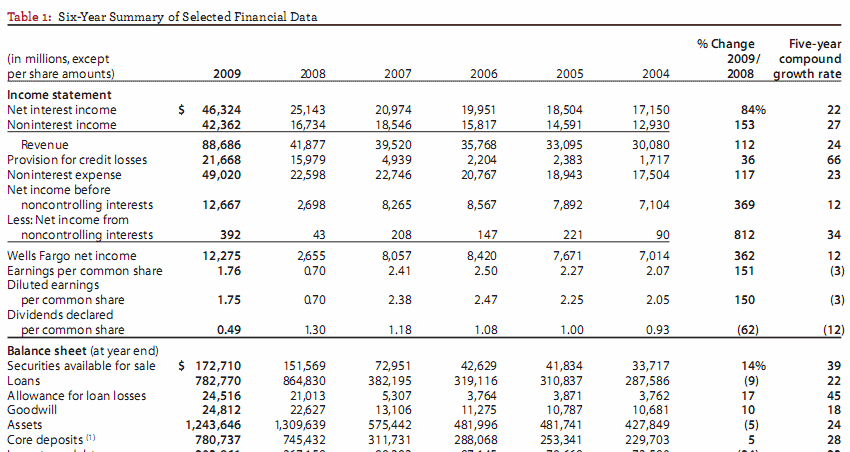This blog post describes how to insert qualifers to make "text to columns" conversion easier.

Example

I copied a table from Wells Fargo annual report (pdf), see image above. Paste it into an excel sheet.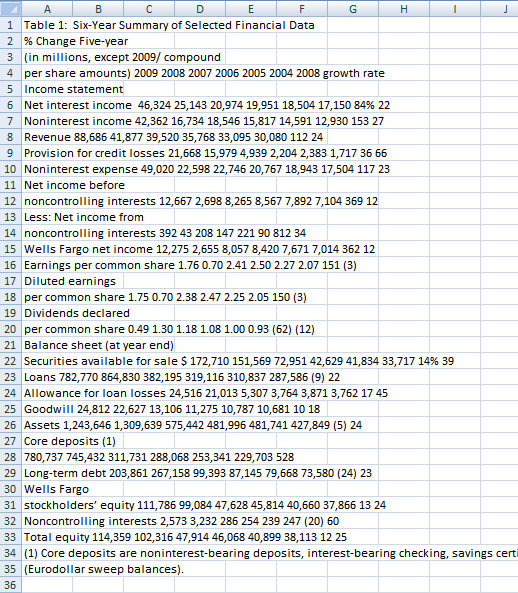I then used "Text to Columns":

1. Select column A
2. Go to tab "Data"
3. Press with left mouse button on "Text to Columns" button.

I got the following result, see image below. Each word is split into a column each, this is not what is wanted.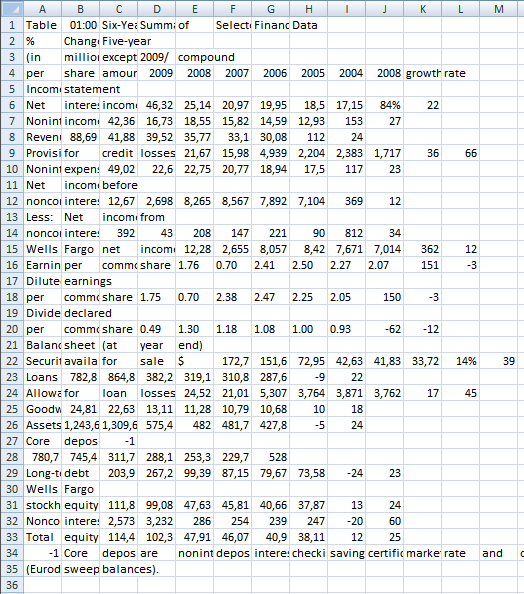The table is a mess, however, the "text to columns" wizard allows you to select a text qualifer to convert words into a single cell.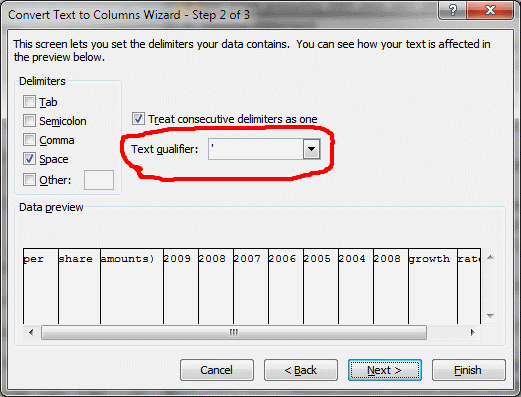This custom function inserts ' (apostrophe) before and after text. The rules are if a character is a number and the next character is a letter then insert an apostrophe before the letter.

The same thing if a character is a letter and the next character is number then insert a apostrophe before the number.

```Function Ins_text_qualifiers(Str)
Dim TLen As Long
Dim i As Long
Dim j As Long
TLen = Len(Str)Str = Trim(Str)
For i = 1 To TLen
If j = TLen + 1 Then Exit For
If Asc(Mid(Str, i, 1)) >= 65 And Asc(Mid(Str, i, 1)) <= 90 _
Or Asc(Mid(Str, i, 1)) >= 97 And Asc(Mid(Str, i, 1)) <= 122 Then
Str = Left(Str, i - 1) & "'" & Mid(Str, i, TLen)
i = i + 1
For j = i To TLen
If Asc(Mid(Str, j, 1)) >= 48 And Asc(Mid(Str, j, 1)) <= 57 Then
Str = Left(Str, j - 2) & "'" & Mid(Str, j - 1, TLen)
i = j
Exit For
End If
Next j  End IfNext i
If Asc(Mid(Str, Len(Str), 1)) >= 65 And Asc(Mid(Str, Len(Str), 1)) <= 90 _
Or Asc(Mid(Str, Len(Str), 1)) >= 97 And Asc(Mid(Str, Len(Str), 1)) <= 122 Then Str = Str & "'"
Ins_text_qualifiers = Str
End Function
```

### Example

per share amounts) 2009 2008 2007 2006 2005 2004 2008 growth rate

becomes

'per share amounts) ' 2009 2008 2007 2006 2005 2004 2008 'growth rate'

### The final result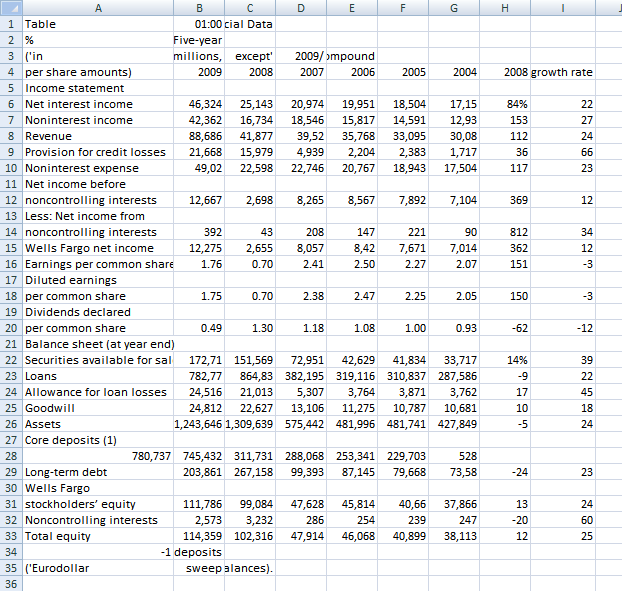It is not perfect but it is a lot better.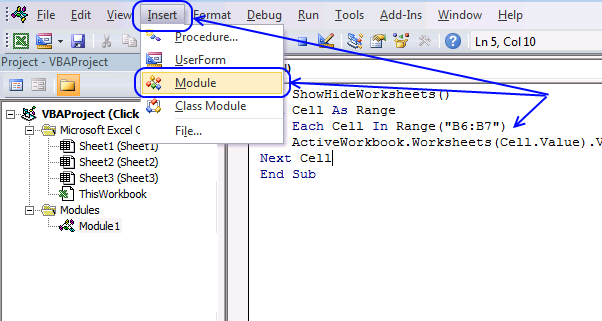(The macro shown in the image above is not used in this article, it only shows you where to paste the code.)

1. Press Alt-F11 to open the visual basic editor
2. Press with left mouse button on Module on the Insert menu
3. Copy and paste the above user defined function
4. Exit visual basic editor
5. Select a cell (B1)
6. Type =Ins_text_qualifiers(A1) into formula bar and press ENTER

### Text to columns (Excel 2007)

1. Select "Data" tab on the ribbon
2. Press with left mouse button on "Text to columns" button

### Get the Excel filetext-qualifier1.xls